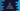# Different ways in C# to find the factorial of a number## Different ways in C# to find the factorial of a number:

In this post, we will learn how to find the factorial of a number in C#. We will write one C# program that will take one number as input from the user and print out the factorial for that number.

Factorial of a number is the multiplication of all numbers from 1 to that number. For example, factorial of 5 is 12345. Finding out a factorial can be done in different ways in C#. We will check these methods to solve it.

### Method 1: Using for loop:

Using a for loop, we can find the factorial of a number easily. For example, let’s take a look at the program below:

``````using System;

public class Program
{
public static void Main()
{
int givenNumber, factorial = 1;

Console.WriteLine("Enter a number to find the factorial ");

for(int i = 2; i<= givenNumber; i++){
factorial *= i;
}

Console.WriteLine("Factorial : {0}",factorial);

}
}``````

Here,

• we have created two variables givenNumber and factorial. givenNumber is used to hold the user given number and factorial to hold the final factorial value.
• We are reading the user input number and storing it in the variable givenNumber.
• Using a for loop, we are iterating from i = 2 to i = givenNumber. i.e. we are iterating all numbers from 2 to that number.
• For each value of i, we are multiplying it with factorial. The initial value of factorial is 1.
• Finally, we are printing the value of factorial.

### Sample Output:

It will print outputs as like below:

``````Enter a number to find the factorial
4
Factorial : 24

Enter a number to find the factorial
10
Factorial : 3628800``````

### Method 2: Using a different function:

We can put the factorial part in a different function and call that function directly. For example:

``````using System;

public class Program
{
static int getFactorial(int number){
int factorial = 1;
for(int i = number; i > 1; i--){
factorial = factorial * i;
}
return factorial;
}

public static void Main()
{
int givenNumber;

Console.WriteLine("Enter a number to find the factorial ");

Console.WriteLine("Factorial : {0}",getFactorial(givenNumber));

}
}``````

This time, I kept everything same, only the factorial part is moved to a different function. This function, getFactorial, takes one number as argument and returns the factoral value. We are using a for loop to find the factorial value in this method.

### Method 3: Using while loop:

We can use one while loop to do the same thing. The while loop will run from number to 2 in reverse order and multiply all values to find the factorial. Let’s write the program:

``````using System;

public class Program
{
static int getFactorial(int number){
int factorial = 1;

while(number > 1){
factorial = factorial * number;
number--;
}
return factorial;
}

public static void Main()
{
int givenNumber;

Console.WriteLine("Enter a number to find the factorial ");

Console.WriteLine("Factorial : {0}",getFactorial(givenNumber));

}
}``````

This program will give output as like the previous one:

``````Enter a number to find the factorial
5
Factorial : 120``````

### Method 4: Recursive approach:

We can also solve it by calling a method recursively. Recursive method calls itself again and again until an end point is reached. The below program shows how to find the factorial recursively in C#.

``````using System;

public class Program
{
static int getFactorial(int number){
if(number == 1){
return 1;
}
return number * getFactorial(number - 1);
}

public static void Main()
{
int givenNumber;

Console.WriteLine("Enter a number to find the factorial ");